Courses

# Work Done Calculation and Adiabatic Expansion and Compression (Reversible and Irreversible) Class 11 Notes | EduRev

## Class 11 : Work Done Calculation and Adiabatic Expansion and Compression (Reversible and Irreversible) Class 11 Notes | EduRev

The document Work Done Calculation and Adiabatic Expansion and Compression (Reversible and Irreversible) Class 11 Notes | EduRev is a part of the Class 11 Course Chemistry Class 11.
All you need of Class 11 at this link: Class 11

WORKDONE CALCULATION

(1) Isochoric process:

V = constant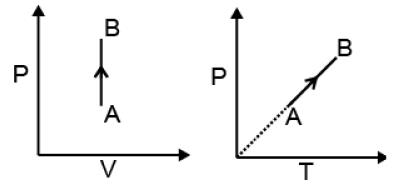dV = 0

 W = 0

dU = dqV
ΔU = q = nCVΔT

 ΔH = nCpΔT

(2) Isobaric process:
W = - Pext (V2-V1)
Reversible & isobaric process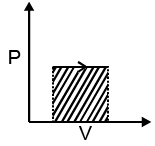W = - P (V2-V1)
= - nR (T2-T1)
Irreversible & isobaric process
P1 = P2 = Pext
For reversible & irreversible isobaric or isochoric process, workdone is same.

(3) Isothermal process.

(a) Reversible Expansion or compression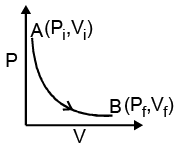W = -P∫ dv
= -∫Pgas dV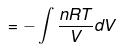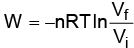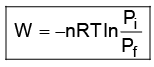In Expansion W = - ve
ΔE = 0

 q = -W

(b) Single stage irreversible expansion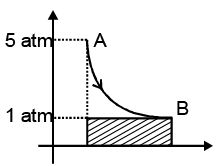W = - Pext(V2 - V1)

|Wrev| > |Wirr| (in case of expansion)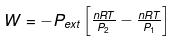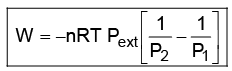(c) Two Stage irreversible Expansion:

Stage I. Pext  = 3 atm, Pi = 5 atm
Stage II. P"ext  = 2 atm , Pf = 2 atm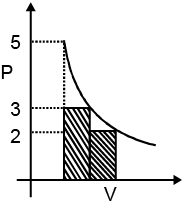Workdone in 2nd stage > Workdone in Ist stage

(d)  n- stage expansion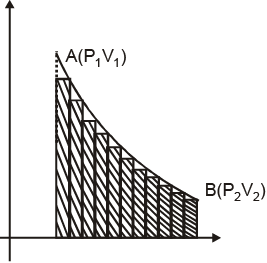Compression - (One stage Compression )

| Wirr | = Pext DV
P1 = 1 atm , P2 = 5 atm , Pext = 5 atm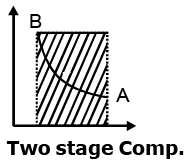| Wirr | > | Wrev | For compression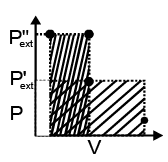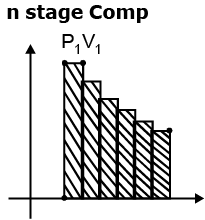Example 1. 2 moles of an ideal gas initially present in a piston fitted cylinder at 300 K, and 10 atm are allowed to expand against 1 atm but the piston was stopped before it stablished the mechanical equilibrium. If temperature were maintained constant through out the change and system delivers 748.26 J of work, determine the final gas pressure and describe the process on PV diagram.
Solution.
Wirrv = - 748.26
Wirr = - Pext [1/P2- 1/P1]nRT
P2 = 4atm

Example 2. 1150 Kcal heat is released when following reaction is carried out at constant volume.
C7H16(l) 11O2(g) → 7CO2(g) 8H2O(l)
Find the heat change at constant pressure.
The pressure of liquid is a linear function of volume (P = a + bV) and the internal energy of the liquid is U = 34 + 3PV find a, b, w, ΔE & ΔH for change in state from 100 Pa, 3m3 to 400 Pa, 6m3

Solution. 100 = a + bV
⇒ 100 = a + 3b
Also, 400 = a + 6b
⇒ a = -200, b = 100
ΔU = 34 + 3(P2V2 - P1V1)
= 6300 J
ΔH = ΔU + P2V2 - P1V1
= 6300 + 2100 = 8400 J
P is a linear function
Pext = (400 + 100)/2 = 250
W = - Pext(dV)
= - 250(6 - 3) = - 750 J

Example 3. 4 moles of an ideal gas (Cv = 15 J) is subjected to the following process represented on P - T graph. From the given data find out whether the process is isochoric or not ? also calculate q, w, ΔU, ΔH,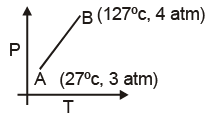Solution. PV = nRT
4V = 4R × 400
V = 400 R ........(1)
3V = 4R × 300
⇒ V = 400 R ........(2)
i.e., V is constant
w = 0
ΔU = nCV  + ΔT ⇒ 4 ×15 ×100 = 6000 J
ΔH = nCP + ΔT ⇒ n (C+ R ) ΔT
⇒ 4 ×(15 8.3)×100
⇒ 9320 J

 q = ΔU = 6kj

Example 4. 2 mole of a gas at 1 bar and 300 K are compressed at constant temperature by use of a constant pressure of 5 bar. How much work is done on the gas ?
Solution.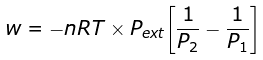= 19953.6 J

Example 5. 2 moles of an ideal diatomic gas (C = 5/2 R) at 300 K, 5 atm expanded irreversibly and adiabatically to a final pressure of 2 atm against a constant pressure of 1 atm.
(1) Calculate final temperature q, w, ΔH & ΔU
(2) Calculate corresponding values if the above process is carried out reversibly.
Solution.
w = C(T2 - T1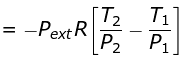Given T2 = 270K
Pext = 1,P2 = 2,P= 5
q = 0
w = ΔU = nCVΔT
= -1247.1 J
ΔH = nCPΔT
= 1745.94 J
If process is reversible Pvγ = Constant
P1- γTγ  = Constant

 T = 231 K

ADIABATIC IDEAL GAS EXPANSION AND COMPRESSION

dq = 0
dU = dW

 Δ U = W

 W = nCV ΔT = nCV T2 - T1)

For an ideal gas CP - CV = R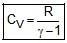nCVdT = - Pext dV
Pint = dP = Pext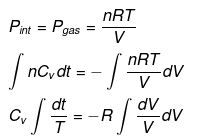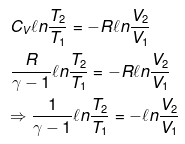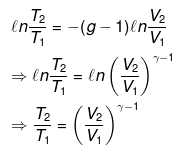⇒ T2(V2)γ - 1 = V1γ - 1T1

TVγ - 1 = Constant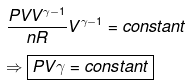dU = dW

⇒ nCV(T2 - T1) = - Pext dV

= - Pext [V2 - V1]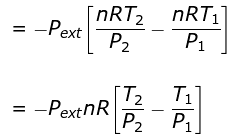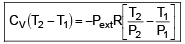COMPARISON OF REVERSIBLE ISOTHERMAL AND REVERSIBLE ADIABATIC IDEAL GAS EXPANSION
(i) If final volumes are same
Isothermal process.
P1v1 = Piso V
⇒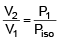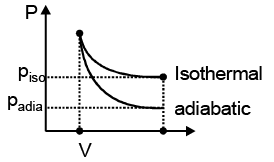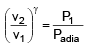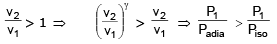(ii) If final pressures are same
Isothermal process.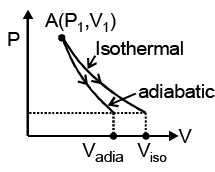P1V1 = P2 Viso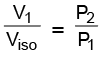.......(1)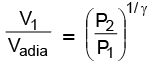In ideal gas expansion,
| Wiso | > | Wadia |

Hence

Compression

(i) If final volumes are same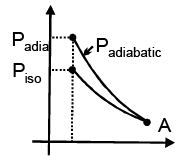For isothermal process

P1V1 = PisoV2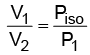..........(1)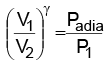..........(2)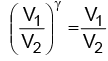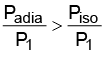(ii) If final pressures are same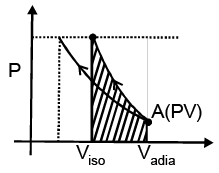P1V1 = PViso ..........(1)

⇒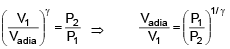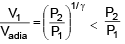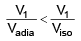Offer running on EduRev: Apply code STAYHOME200 to get INR 200 off on our premium plan EduRev Infinity!

## Chemistry Class 11

136 videos|245 docs|180 tests

,

,

,

,

,

,

,

,

,

,

,

,

,

,

,

,

,

,

,

,

,

;